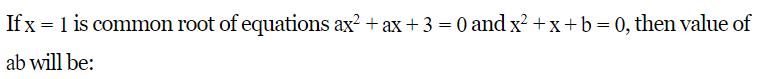# feed

• The speed of a bus increases by 2 km after every one hour. If the distance travelling in the first one hour was 35 km. what was the total distance travelled in 12 hours?

•  A sum of Rs. 10 is given as a loan to be returned in 6 monthly installments at Rs.3. What is the rate of interest?

• In a co-educational school there are 15 more girls than boys. if the number of girls is increased by 10% and the number of boys is also increased by 16% there would be 9 more than boys. what is the number of students in the school?

•